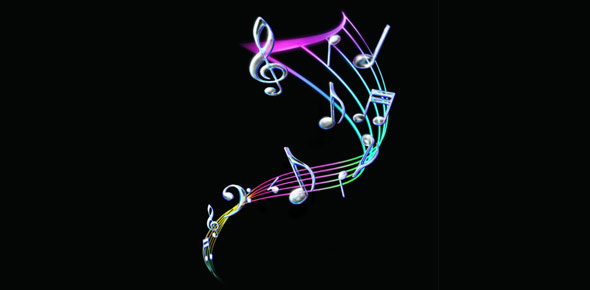# Choir Music Theory Final

Approved & Edited by ProProfs Editorial Team
At ProProfs Quizzes, our dedicated in-house team of experts takes pride in their work. With a sharp eye for detail, they meticulously review each quiz. This ensures that every quiz, taken by over 100 million users, meets our standards of accuracy, clarity, and engagement.
| Written by MissSpeer
M
MissSpeer
Community Contributor
Quizzes Created: 1 | Total Attempts: 1,045
Questions: 12 | Attempts: 1,045SettingsBasic music theory knowledge from Choir class this year.

• 1.

### The time signature is 4/4. How many beats does a quarter note get?

• A.

1

• B.

2

• C.

4

A. 1
Explanation
In a time signature of 4/4, the top number (4) indicates that there are 4 beats in each measure, and the bottom number (4) indicates that a quarter note receives one beat. Therefore, a quarter note gets one beat in a 4/4 time signature.

Rate this question:

• 2.

### The time signature is 6/8. How many beats are in each measure and what note gets one beat?

• A.

Eight beats per measure and a 6th note gets one beat.

• B.

Six beats per measure and an 8th note gets one beat.

• C.

Four beats per measure and the quarter note gets one beat.

B. Six beats per measure and an 8th note gets one beat.
Explanation
The time signature 6/8 indicates that there are six beats in each measure. The top number, 6, represents the number of beats per measure, while the bottom number, 8, indicates that an eighth note receives one beat. Therefore, in this time signature, there are six beats per measure and an eighth note gets one beat.

Rate this question:

• 3.

### Assume that C is your Do. What note is the Fa?

• A.

E

• B.

F

• C.

F#

B. F
Explanation
If C is considered as Do, then the note that comes after C is D, and the note that comes after D is E. So, if C is Do, then the note that is two steps higher than C is E. Therefore, the note that is three steps higher than C (Fa) would be F.

Rate this question:

• 4.

### Assume that G is your Do. What note is the Sol?

• A.

C

• B.

E

• C.

D

C. D
Explanation
If G is your Do, then the note that is the Sol would be D. In the solfege system, the note that corresponds to the sol is always a perfect fifth above the Do. Since G is the Do in this case, the note a perfect fifth above G would be D. Therefore, D is the correct answer.

Rate this question:

• 5.

### True or False? Whole Whole Half Whole Whole Half is the correct way to figure out the notes in any given key.

• A.

True

• B.

False

B. False
Explanation
The given answer is false because the correct way to figure out the notes in any given key is by following the pattern of Whole Whole Half Whole Whole Whole Half. This pattern represents the intervals between the notes in a major scale, which is the basis for determining the notes in a key. The pattern of Whole Whole Half is incomplete and does not accurately represent the intervals in a major scale. Therefore, the statement that Whole Whole Half Whole Whole Half is the correct way to figure out the notes in any given key is false.

Rate this question:

• 6.

### Which of the following markings are actual music symbols?

• A.

Mp

• B.

Mf

• C.

F

• D.

Pp

• E.

Vf

• F.

Sfz

• G.

R.

• H.

Ff

A. Mp
B. Mf
C. F
D. Pp
F. Sfz
H. Ff
Explanation
The given markings are all actual music symbols used to indicate dynamics (the volume or intensity of the music). "mp" stands for mezzo-piano (moderately soft), "mf" stands for mezzo-forte (moderately loud), "f" stands for forte (loud), "pp" stands for pianissimo (very soft), "sfz" stands for sforzando (strong accent), and "ff" stands for fortissimo (very loud).

Rate this question:

• 7.

### What are the notes that go in spaces on a treble (G) clef?

• A.

FACE

• B.

ABCD

• C.

ACEG

A. FACE
Explanation
The correct answer is FACE because in music notation, the spaces on a treble clef staff are filled with the notes F, A, C, and E. Each letter represents a different pitch, and when played in sequence, they create a specific melody or harmony. Therefore, FACE is the correct answer for the notes that go in spaces on a treble clef.

Rate this question:

• 8.

### What are the notes that on on the lines on a treble (G) clef?

• A.

DFACG

• B.

FACEG

• C.

EGBDF

C. EGBDF
Explanation
The notes that are on the lines of a treble (G) clef are E, G, B, D, and F. This can be remembered using the mnemonic "Every Good Boy Does Fine." Each letter corresponds to a note on the lines of the treble clef starting from the bottom line and moving upwards.

Rate this question:

• 9.

### The interval between Do and Mi is a fourth.

• A.

True

• B.

False

B. False
Explanation
The interval between Do and Mi is actually a third, not a fourth. In a major scale, the interval between the first and third notes is a major third, which is made up of two whole steps or four half steps. Therefore, the correct answer is False.

Rate this question:

• 10.

• 11.

• 12.

### What is the most important thing you learned in choir this year?

Related TopicsBack to top
×

Wait!
Here's an interesting quiz for you.